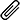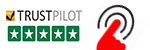High Quality, Fast Delivery, Plagiarism Free - Just in 3 Steps

## Upload Questions Details and Instructions:Select Assignment Files

## Get Assignment Help by Experts

### Plagiarism-Free, Every Time

All of our assignment solution delivers with a dedicated plagiarism report

### Excellent Subject Matter Expert

Get Supreme Assignment Help by highly Skilled Writers

### 100% Satisfaction Guaranteed

Score Higher or Your Money Back# COMP281 C Programming Assignment Help

COMP281 Assignment 1 – C ProgrammingThe following 5 problems will be available as Assignment 1 on the online judging
system available at https://intranet.csc.liv.ac.uk/JudgeOnline/

• You need to write a valid C program that solves each of these problems – it must
read the input, as specified in the problem description then print the solution to the given
problem for that input.
• Input is read from the standard input, in the same way that you read input from the keyboard
as shown in lectures (e.g., using scanf). Output is also printed to the standard output, as
you have seen (e.g., using printf).
• When you are satisfied that your programs work correctly, you must submit them through the
departmental submission system, as described below.
• You must also include a brief report describing your solutions to the problems. This should
be maximum one side of A4 paper (although there is no penalty for a longer report) and
should give a description of how each of your solutions works. This should include
describing the algorithm used to reach the solution, describing your use of any C language
features (that were not discussed in lectures) and identifying any resources that you have used
• This assignment is worth 30% of the total mark for COMP281
• 50% of the marks will be awarded for programs that correctly solve the problem for all test
cases. All problems are weighted equally and each counts for 20% of this total.
• 40% of the marks will be awarded depending on the style, comments and efficiency of the
solution. All problems are weighted equally and each counts for 20% of this total.
• 10% of the marks will be awarded for the quality and depth of the accompanying report
• See separate marking guidelines for more details.
Submission Instructions
• Name each of your c files according to the problem number; i.e.,
1014.c,1017.c,1025.c,1060.c and 1030.c. Place all five of your C files and your
report (in .pdf format) into a single zip file.
• All question have also been/will also be posted on www.csc.liv.ac.uk/JudgeOnline
• Before you submit your solutions, please first test them using the online judge. You
are required to include the “Result” and the “RunID” in your C code as comments.
The OJ provides a RunID. RunIDs are not problem IDs.
! Example: the problem is 10xx. The solution has been accepted by the OJ,
and the runID is 2033. You add to your code: /* 2033 Accepted */
! Result is one of the following: Accepted, Wrong Answer, Presentation Error,
Time Limit Exceeded, Memory Limit Exceeded, Output Limit Exceeded,
Run time Error, Compile Error
• Submit this zip file using the departmental submission system at

https://www.csc.liv.ac.uk/cgi-bin/submit.pl

Only the file submitted through this link will be marked

• The deadline for this assignment 20/02/2015, 5:00pm
• Penalties for late submission apply in accordance with departmental policy as
set out in the student handbook, which can be found at:

https://www.csc.liv.ac.uk/student/ugpdfhandbook.pdf

Problem 1014
Title: Area and circumference of circles

Description:
In this exercise you have to compute the area and circumference of a series of circles and output their sum.
Specifically, the program will take two radii of two circles as input (r1 <= r2, both integers) and will output the sum of
the areas and the circumferences of all circles starting with r1 and increasing at each step the radius by ‘1’ until radius
r2 has been reached. As an example, suppose ‘r2-r1 = 2′ then the program has to compute the sum of the areas and
circumferences of three circles with radii r1,r1+1,r2
Remember that the area of a circle equals Pi*r^2 and the circumference equals 2Pi*r.
Set Pi to 3.14
Input:
Two
integers
r1
and
r2
with
r1<=r2
Output:
two floats, sum_of_areas and sum_of_circumferences.
Sample Input:
3 4
Sample Output:
78.500000
43.959999
Problem 1017
Title: Product of integers in columns
Description:
In this exercise you have to write a program that can compute the product of the numbers (integers in this case) in a
series of columns.
Input:
The first number of the input, N, describes the number of columns that will follow, then N columns follow. The first
row will contain the first integer of each column. This number M will indicate how many numbers will follow in the
respective column. Note that this means that not each column needs to be of the same length.
Output:
N numbers that represent the product of each column.
Sample Input:
4
3 2 3 4
1 1 1 2
2 3 2 2
3 4 3
2
Sample Output:
6 3 8 24
Problem 1025
Title: Largest common factor and smallest common multiple
Description:
Input two positive integers. Compute their largest common factor and smallest common multiple.
Input:
Input two positive integers.
Output:
largest common factor and smallest common multiple.
Sample Input:
2 3
Sample Output:
1 6
Problem 1060
Title: Sort even integers
Description:
From the input of 10 numbers, sort only the even integers into ascending order.
Print the list with all odd numbers (including 0) in their corresponding place in the input and the even numbers sorted
into ascending order.
Input:
10 integers separated by spaces. (All integers are at most 32 bits)
Output:
An evenly sorted list (even numbers in ascending order, odd numbers unchanged)
Sample Input:
5 3 4 2 1 6 8 7 10 9
Sample Output:
1 3 2 4 5 6 8 7 10 9
Sample Input 2:
11 16 14 12 10 8 6 4 2 1
Sample Output 2:
11 2 4 6 8 10 12 14 16 1
Problem 1030
Title: Precise Division
Description:
8/13=0.615384615384615384615384…
For 8/13, the 5-th digit after the decimal point is 8.
Given three positive integers a, b, and n (all at most 60000), you are asked to compute a/b and print out the n-th digit
after the decimal point.
Input:
a b n (three positive integers)
Output:
The n-th digit after the decimal point of a/b
Sample Input:
8 13 5
Sample Output:
8We're here to instantly help! Get best assignment questions and answers help 24/7 and Earn better grades with homework